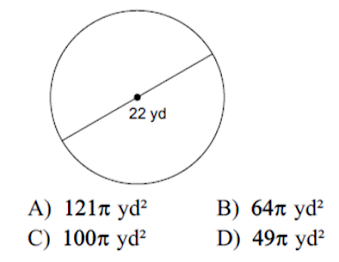Section 4 test - polygon area and perimeter
Last Name *
Period *
Find Area of trapezoid *Find Area *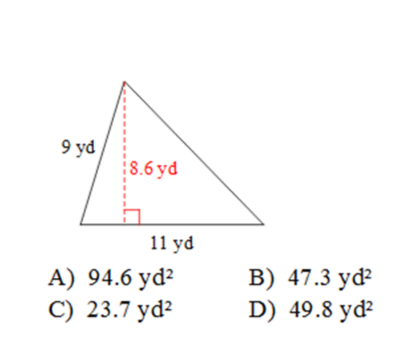Find the area of a trapezoid with bases of 14 and 22, and a height of 9 *
Find the area of a 45-45-90 triangle with legs of 8 *
Find the area of a equilateral triangle with sides of 8 *
Find the area of a circle with a diameter of 7. Use 3.14 for pi *
Find area of parallelogram *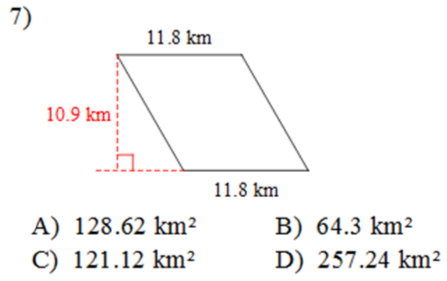*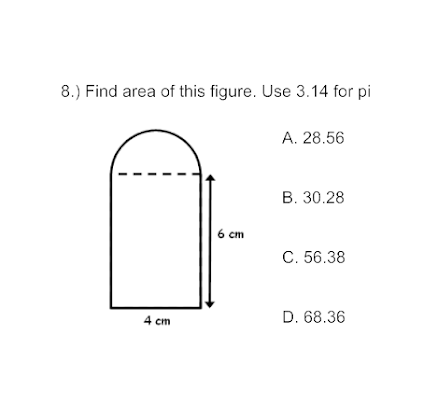Find Perimeter *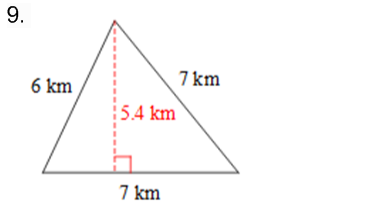Find Perimeter *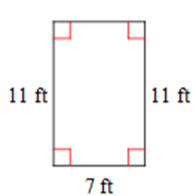Remember that the formula for a triangle has a divide by 2 in it *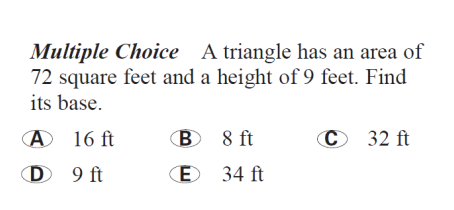Find the area of the shaded region, use 3.14 *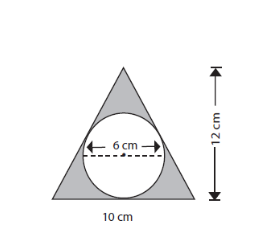Find the area of the parallelogram *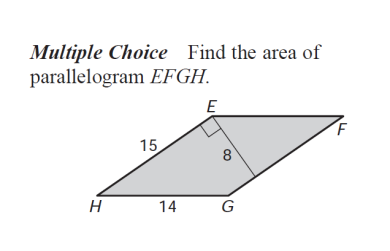Find the area of the square inscribed in a circle. The circle has a diameter of 16. *
Area of a circle inscribed in a square. Use 3.14 *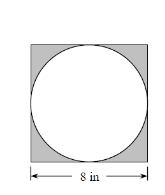Find area of the special right triangle *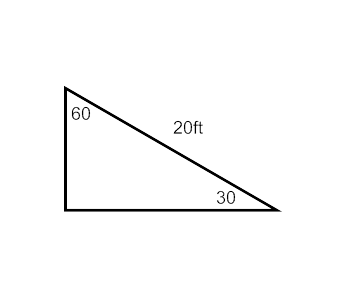A rectangle has a width to length ratio of 3:4. What are side lengths if the area is 588 *
*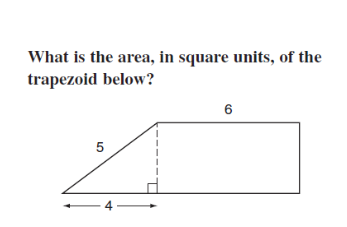*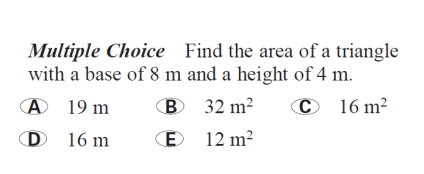Find length *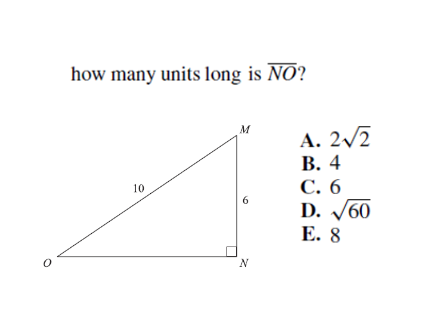Find area of square *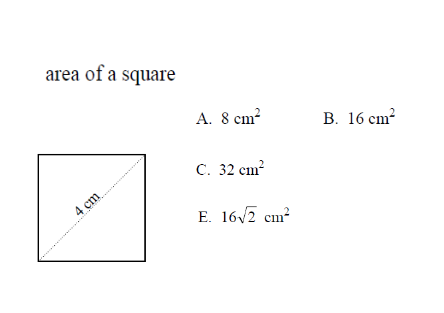Rectangle word problem *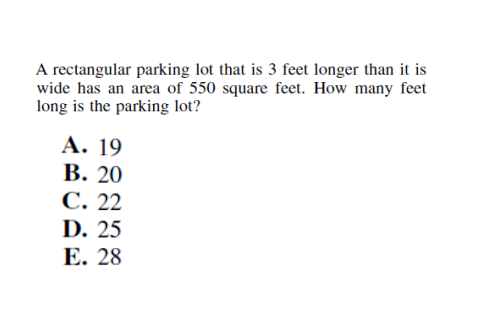Use the trapezoid formula *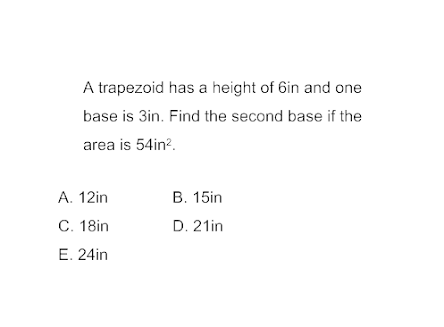find Area *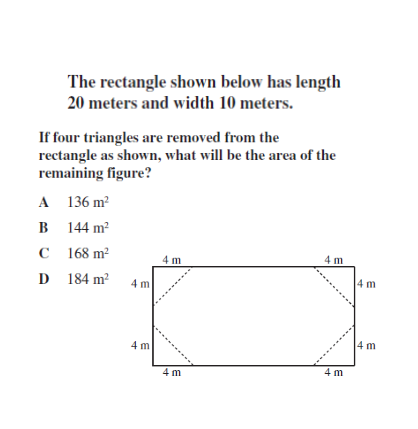Find area of parallelogram *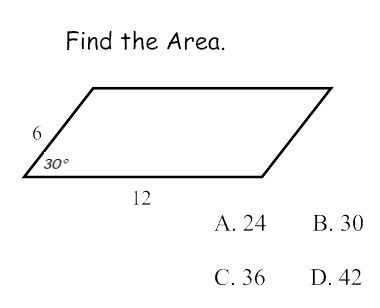Find area of the triangle *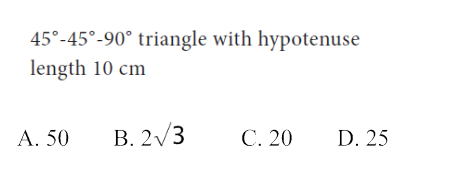Area of an isosceles triangle with two sides of 20 and a base of 24 *
Find area of the trapezoid *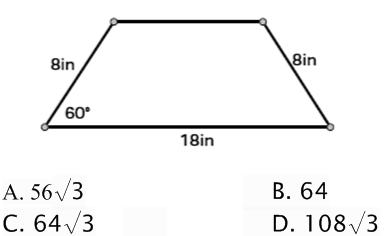Find perimeter of the trapezoid *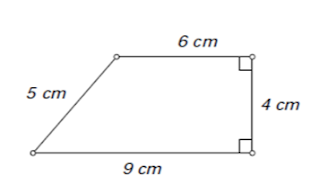Find the area of the triangle *Find the total area of this compound shape *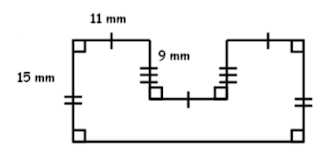Find the area of trapezoid *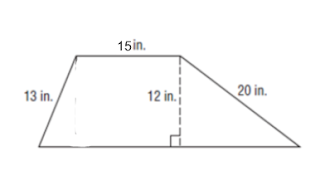The polygon below was a rectangle and then the triangle has been cut out. Find the PERIMETER of the remaining figure after the cut out. *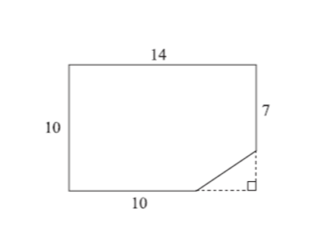Find the exact area of the circle *